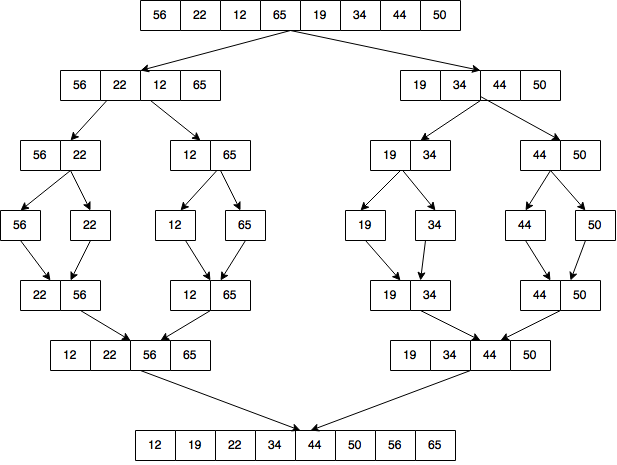# Computer Science : Mergesort

## Example Questions

### Example Question #2 : Sorting

How is merge sort accomplished?

If there are an even number of elements, the list is broken into two groups, are sorted, then merged back together. If there are odd numbered elements, the list is broken into three groups.

The original list is continuously broken up into sublists until each sublist is containts 1 element, then the sublists are combined together.

The original list is broken into two groups, then sorted from there.

The orginal list is broken into sublists of 4, then are combined together.

Each element in the list is compared to all the other elements and inserted where it fits.

The original list is continuously broken up into sublists until each sublist is containts 1 element, then the sublists are combined together.

Explanation:

In merge sort, a list is broken up into sublists containing 1 element. Each element is then compared to another element and sorted. Each 2-element group is then combined with other 2-element groups, comparing the first value of each group and deciding how to four elements. The larger group of four is compared to another group of four, until the process ends and the list is sorted.

### Example Question #1 : SortingThe above diagram represents what type of sorting algorithm?

Mergesort

Insertion sort

Selection sort

Quicksort

Bubblesort

Mergesort

Explanation:

Mergesort consists of breaking down an unsorted list into subarrays; sorting each sub-array, and recombining the sub-arrays into larger arrays.

### Example Question #1 : Sorting

Of the choices below, what is the most efficient sorting algorithm for an unordered list where the size of the list is an odd number and the size of the list is finite?

Insertion Sort

Mergesort

Bubble Sort

Selection Sort